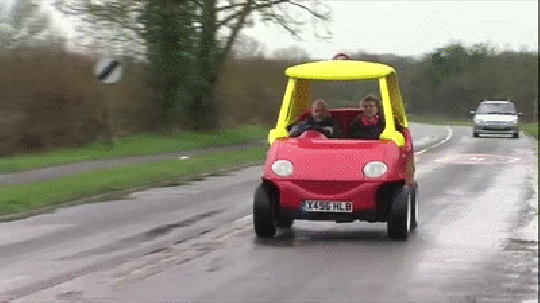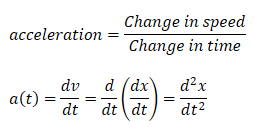top of page
Search

# Kinematics TutorialKey Concepts: Displacement Velocity Acceleration Equations of motion Projectile motion QUESTION 1: "A car is driving down a highway. The attached graph shows its displacement as a function of time. Determine its speed and its equation of motion."How do we figure out the speed of the car just by looking at this graph? It's actually pretty simple. Think about it like this: If it takes me 1 hour to drive between two cities that are 100 km away from each other, then clearly my speed will have been:So basically we need 2 things from the graph: How far the car has gone and how much time it spent going this far. To do this, we look at how much the graph has "gone up" compared to how much it has "gone forward".:Very nice. To determine the equation of motion, we write:QUESTION 2: "A car is driving down a highway. The attached graph shows its speed as a function of time. Determine its acceleration and its equation of motion."Ok, so this time the task is a little different. We need to find the "acceleration" of the car. What is "acceleration" anyways? Let's make a small drawing:Basically, we can say that the car is accelerating because it speed keeps changing. In this case the speed keeps increasing so the acceleration must be positive. We can define acceleration as:The acceleration is 3m/s^2. This means that for every second that passes, the speed increases by 3m/s. We still need the "Equation of motion". How do we find that? In the first question, we found thatBasically we can find the speed by using calculus to take the derivative of the distance. We can do the same thing for the acceleration:Here is the point: "If we have x(t), we can find v(t) and a(t) by simply taking derivatives!" Ok, but in this situation, we have the a(t)=3m/s^2. We can find x(t) by doing the opposite of taking the derivative: Taking the integral!Let's try to graph it:Weird. The displacement graph is sort of bending upwards. I mean that kind of makes sense. The car is accelerating so it's going faster and faster. In the first 4 seconds, the car is not going very fast, so it only covers 25m. In the next 4 seconds it covers 75m. We can write the General equation of motion:^This works if acceleration is constant. QUESTION 3: "A ball is thrown sideways at a speed of 3m/s from a 100m tall building. How far from the base of the building does it hit the ground?" Let's make a sketch to figure out what is going on:In the beginning, the ball is just flying straight to the right because of its initial speed. However, gravity pulls it down and gives it a vertical speed. After a certain amount of time, it hits the ground a distance d away from the building. Here is a useful trick we can use: "We can write down 2 equations of motion. One for motion in the horizontal x-direction and one for the vertical y-direction." How should we do this for the current case? The initial speed of the ball in the x-direction is 3m/s. No force is pulling the ball to the right, so there is no acceleration. For the y-direction, the ball starts at y=h and gets pulled down by gravity. The acceleration it experiences is a=-9.82 m/s^2. So in total we can write:Very nice. This is a good start. We need to find the distance at which the ball hits the ground. We know that it hits the ground after a certain number of seconds. Let us write:Here "ti" is the "impact time". We already know v0 and we want to find d. So can we find an expression for ti somehow? Take a look at the y-equation. When y=0 we know that the ball has hit the ground. We can therefore write:^So the impact time depends on how far up we start and how much the ball accelerates due to gravity. Let's plug this into the expression for the impact distance:The ball hits the ground 13.4m away from the base of the building.